# Texas Go Math Grade 4 Lesson 1.3 Answer Key Compare and Order Numbers

Refer to our Texas Go Math Grade 4 Answer Key Pdf to score good marks in the exams. Test yourself by practicing the problems from Texas Go Math Grade 4 Lesson 1.3 Answer Key Compare and Order Numbers.

## Texas Go Math Grade 4 Lesson 1.3 Answer Key Compare and Order Numbers

Unlock the Problem

Grand Canyon National Park in Arizona had 651,028 visitors in June and 665,188 visitors in July. In which month did the park have more visitors?

How many visitors were there in June?
It is given that
Grand Canyon National Park in Arizona had 651,028 visitors in June and 665,188 visitors in July
Hence, from the above,
We can conclude that
The number of visitors in June are: 651,028

How many visitors were there in July?
It is given that
Grand Canyon National Park in Arizona had 651,028 visitors in June and 665,188 visitors in July
Hence, from the above,
We can conclude that
The number of visitors in July are: 665,188

Share and Grow

Question 1.
Compare 145,158,190 and 145,185,170.
Write <, >, or = Use the place-value chart to help.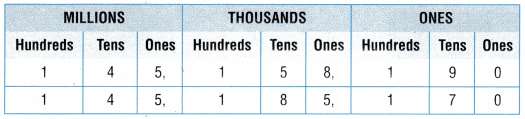145,158,190 _________ 145,185,170
The given numbers are: 145,158,190 and 145,185,170
Now,
The given place-value chart for the given numbers is:Now,
From the above place-value chart,
We can observe that
The Thousand’s period is greater in 145,185,170 than in 145,158,190
Hence, from the above,
We can conclude that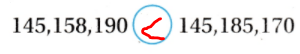Compare. Write <, >, or =.

Question 2.
7,458,562,333 _________ 7,457,541,753
The given numbers are: 7,458,562,333 and 7,457,541,753
Now,
The representation of the given numbers in the place-value chart is: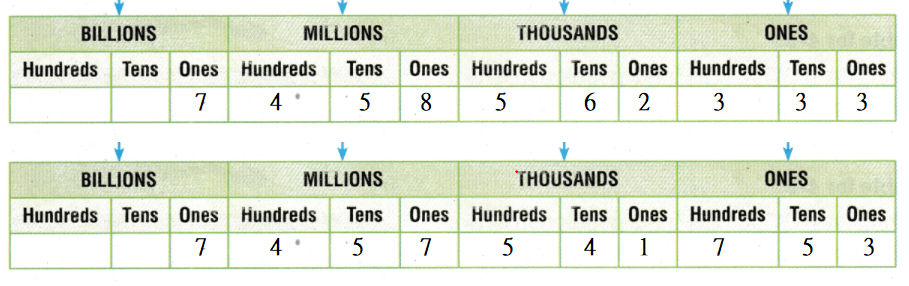Now,
From the above place-value charts,
We can observe that
The Million’s period is greater in 7,458,562,333 than in 7,457,541,753
Hence, from the above,
We can conclude thatQuestion 3.
56,991 _________ 52,880
The given numbers are: 56,991 and 52,880
Now,
The representation of the given numbers in the place-value chart is:Now,
From the above place-value charts,
We can observe that
The Thousand’s period is greater in 56,991 than in 52,880
Hence, from the above,
We can conclude thatQuestion 4.
708,651,515 _________ 629,672,484
The given numbers are: 708,651,515 and 629,672,484
Now,
The representation of the given numbers in the place-value chart is:Now,
From the above place-value charts,
We can observe that
The Million’s period is greater in 708,6511,515 than in 629,672,484
Hence, from the above,
We can conclude thatQuestion 5.
1,430,620 _________ 986,435
The given numbers are: 1,430,620 and 986,435
Now,
The representation of the given numbers in the place-value chart is: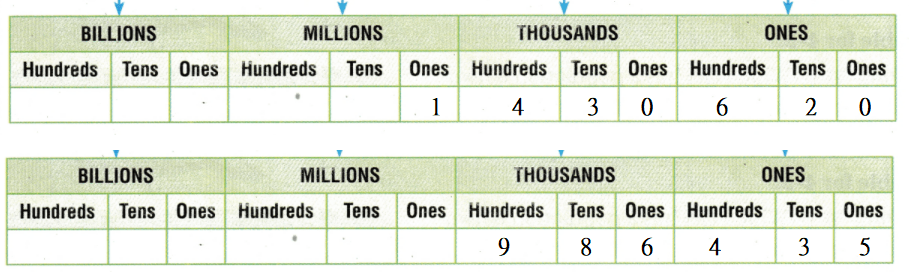Now,
From the above place-value charts,
We can observe that
The Million’s period is greater in 1,430,620 than in 986,435
Hence, from the above,
We can conclude that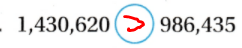Order from greatest to least

Question 6.
20,650; 21,150; 20,890
The given numbers are: 20,650. 21,150, and 20,890
Now,
The representation of the given numbers in the place-value chart is: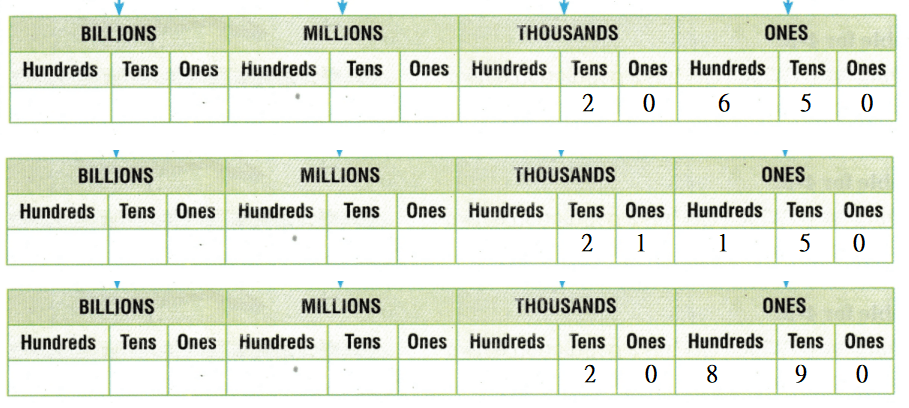Now,
From the above place-value charts,
We can observe that
21,150 > 20,890 > 20,650
Hence, from the above,
We can conclude that
The order of the numbers from the greatest to the least is:
21,150 > 20,890 > 20,650

Problem Solving

H.O.T.
Algebra Write all of the digits that can replace eachQuestion 7.
567 < 55 < 582 ____________
Let the missing digit be x
Now,
The given pattern is:
567 < 5×5 < 582
Now,
From the above pattern,
We can observe that
The ten’s place-value in the given pattern is increasing
So,
The missing number should be: 7
Hence, from the above,
We can conclude that
The completed pattern is:
567 < 575 < 582

Question 8.
3,408 < 3,30 < 3,540 ____________
Let the missing digit be x
Now,
The given pattern is:
3,408 < 3,x30 < 3,540
Now,
From the above pattern,
We can observe that
The hundred’s place-value in the given pattern is increasing and then becomes constant
So,
The missing number should be: 5
Hence, from the above,
We can conclude that
The completed pattern is:
3,408 < 3,530 < 3,540

Use the pictograph for 9-11.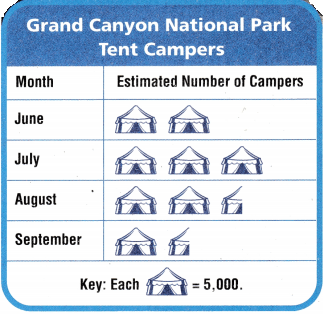Question 9.
In which month shown did the Grand Canyon National Park have about 7,500 tent campers?
The given pictograph is:Now,
From the above pictograph,
We can observe that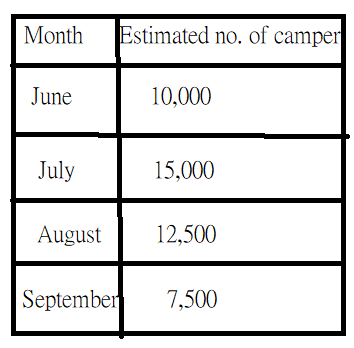Hence, from the above,
We can conclude that
September month shows that the Grand Canyon National Park has about 7,500 tent campers

Question 10.
Which months had more than 10,000 tent campers?
The given pictograph is:Now,
From the above pictograph,
We can observe thatHence, from the above,
We can conclude that
July and August months had more than 10,000 tent campers

Question 11.
What if during the month of October, the park had 22,500 tent campers? How many symbols would be placed on the pictograph for October?
It is given that
During the month of October, the park had 22,500 tent campers
Now,
The given pictograph is:So,
From the above pictograph,
We can observe that
The number of symbols that would be placed on the pictograph for October = $$\frac{22,500}{5,000}$$
= 4.5
Hence, from the above,
We can conclude that
The number of symbols that would be placed on the pictograph for October is: 4 full symbols + $$\frac{1}{2}$$ symbol

Question 12.
H.O.T. What’s the Question?
Compare: 17,643,251; 17,633,512; and 17,633,893. The answer is 17,633,512,
The given numbers are: 17,643,251, 17,633,512, and 17,633,893
Now,
The representation of the given numbers in the place-value chart is:Now,
From the above place-value chart,
We can observe that
The order of the numbers from the greatest to the least is:
17,643,251 > 17,633,893 > 17,633,512
Hence, from the above,
We can conclude that
The question required for this problem is:
What is the least number?

Question 13.
Multi-Step What’s the Error?
Max said that 36,594,145 is less than 5,980,251 because 3 is less than 5. Describe Max’s error and give the correct answer.
It is given that
Max said that 36,594,145 is less than 5,980,251 because 3 is less than 5
Now,
The given numbers is: 36,594,145 and 5,980,251
Now,
The representation of the given numbers in the place-value chart is:Now,
From the above place-value chart,
We can observe that
The Million’s period is greater i.e., 3 > 0
Hence, from the above,
We can conclude that
Max’s error is: Misplacement of digits in the place-value chart
36,594,145 > 5,980,251

Question 14.
The hotter a pepper, the higher the number of units it is rated. The Scotch bonnet pepper isn’t as hot as a Fatali pepper. Which could be the rating of the Scotch bonnet pepper?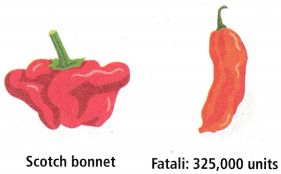(A) 325,500 units
(B) 400,000 units
(C) 330,000 units
(D) 300,000 units
It is given that
The hotter a pepper, the higher the number of units it is rated. The Scotch bonnet pepper isn’t as hot as a Fatali pepper
Now,
The given rating of Fatali pepper is: 325,000 units
Now,
According to the given information,
The rating of Scotch bonnet pepper will be less than the rating of Fatali pepper i.e., less than 325,000 units
Hence, from the above,
We can conclude that
The rating of the Scotch bonnet pepper is:Question 15.
Which statement about 3,584,271 and 3,564,092 is true?
(A) 3,584,271 < 3,564,092
(B) 3,584,271 > 3,564,092
(C) 3,584,271 = 3,564,092
(D) 3,564,092 > 3,584,271
The given numbers are: 3,584,271 and 3,564,092
Now,
The representation of the given numbers in the place-value chart is: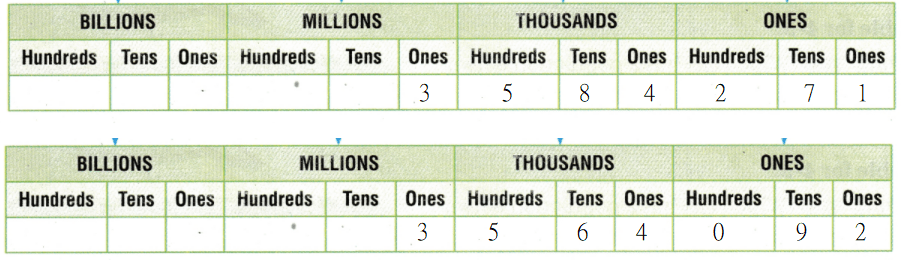So,
From the above place-value chart,
We can observe that
3,584,271 > 3,564,092
Hence, from the above,
We can conclude that
The statement about the given numbers that is true is: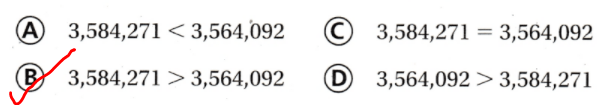Question 16.
Multi-Step The attendance for 3 baseball games was 38,454 people; 38,198 people; and 36,572 people. Which shows the number of people who attended each game written in order from least to greatest?
(A) 36,572 < 38,198 < 38,454
(B) 38,454 < 38,198 < 36,572
(C) 36,572 < 38,454 < 38,198
(D) 38,454 <36,572 <38,198
It is given that
The attendance for 3 baseball games was 38,454 people; 38,198 people; and 36,572 people
Now,
The representation of the given numbers in the place-value chart is:So,
From the above place-value chart,
We can observe that
36,572 < 38,198 < 38,454
Hence, from the above,
We can conclude that
The number of people who attended each game written in order from the least to the greatest is:TEXAS Test Prep

Question 17.
Zachary’s school set a goal of collecting 12,155 cans of food each day. In the first 3 days they collected 12,250 cans; 10,505 cans; and 12,434 cans. Which total was less than their daily goal?
(A) 12,434
(B) 12,250
(C) 12,155
(D) 10,505
It is given that
Zachary’s school set a goal of collecting 12,155 cans of food each day. In the first 3 days they collected 12,250 cans; 10,505 cans; and 12,434 cans
Now,
According to the given information,
The comparison of numbers is:
12,155 < 12,250
12,155 > 10,505
12,155 < 12,434
Hence, from the above,
We can conclude that
The total that was less than their daily goal is: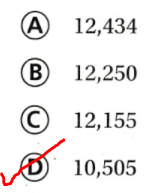### Texas Go Math Grade 4 Lesson 1.3 Homework and Practice Answer Key

Compare. Write <, >, or =.

Question 1.
$6,462 __________$6,471
The given numbers are: 6,462 and 6,471
Now,
The representation of the given numbers in the place-value chart is:Now,
From the above place-value chart,
We can observe that
Ten’s place is greater in 6,462 than in 6,471
Hence, from the above,
We can conclude thatQuestion 2.
453,536 __________ 451,626
The given numbers are: 453,536 and 451,626
Now,
The representation of the given numbers in the place-value chart is:Now,
From the above place-value chart,
We can observe that
Thousand’s place is greater in 453,536 than in 451,626
Hence, from the above,
We can conclude thatOrder from greatest to least.

Question 3.
87,262; 78,529; 78,811
The given numbers are: 87,262, 78,529, and 78,811
Now,
The representation of the given numbers in the place-value chart is: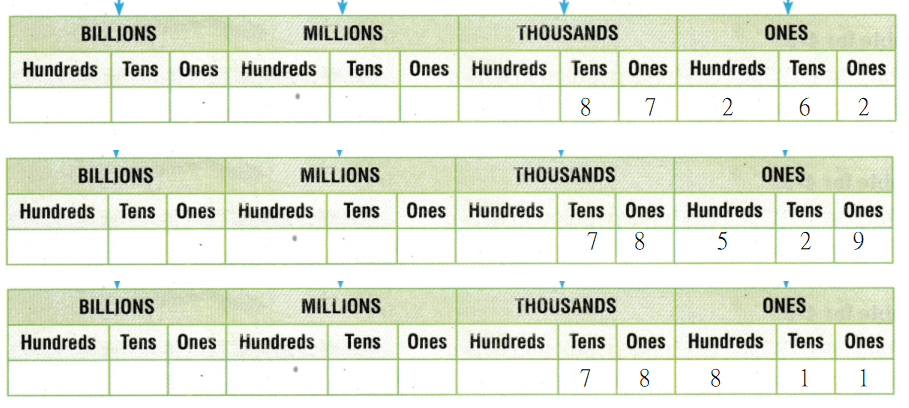Now,
From the above place-value chart,
We can observe that
87,262 > 78,811 > 78,529
Hence, from the above,
We can conclude that
The order of the numbers from the greatest to the least is:
87,262 > 78,811 > 78,529

Question 4.
125,162; 212,225; 128,361
The given numbers are: 125,162, 212,225, and 128,361
Now,
The representation of the given numbers in the place-value chart is: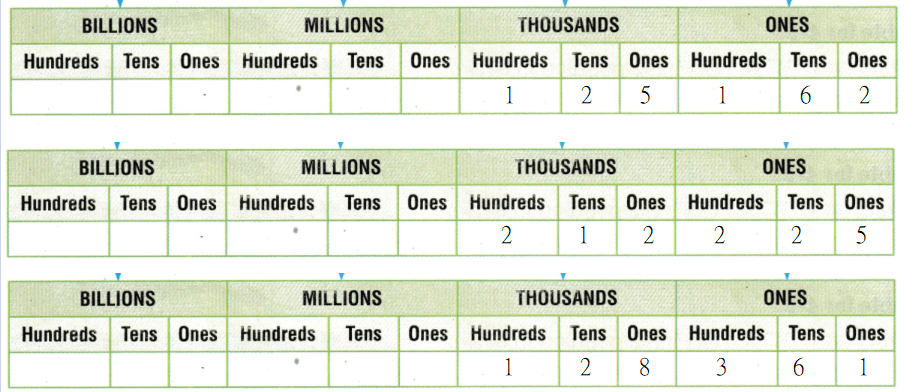Now,
From the above place-value chart,
We can observe that
212,225 > 128,361 > 125,182
Hence, from the above,
We can conclude that
The order of the numbers from the greatest to the least is:
212,225 > 128,361 > 125,182

Write all of the digits that can replace each.
Question 5.
735 < 74 < 761
Let the missing digit be: x
Now,
The given pattern is:
735 < 7×4 < 761
Now,
From the above pattern,
We can observe that
Ten’s place-value is increasing
So,
The missing number should be: 4 (or) 5
Hence, from the above,
We can conclude that
735 < 744 < 761 (or) 735 < 754 < 761

Question 6.
2,307 < 255 < 2,739
Let the missing digit be: x
Now,
The given pattern is:
2,307 < 2,x55 < 2,739
Now,
From the above pattern,
We can observe that
Hundred’s place-value is increasing
So,
The missing number should be: 4 (or) 5 (or) 6
Hence, from the above,
We can conclude that
2,307 < 2,455 < 2,739 (or) 2,307 < 2,555 < 2,739 (or) 2,307 < 2,655 < 2,739

Problem Solving

Use the pictograph for 7-8.Question 7.
During which months did more than 10,000 people attend the circus?
The given pictograph is:Now,
From the above pictograph,
We can observe that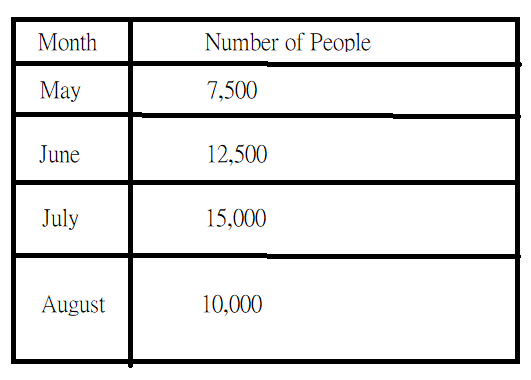Hence, from the above,
We can conclude that
During June and July months, more than 10,000 people attended the circus

Question 8.
List the months in order from greatest attendance to least attendance.
The given pictograph is:Now,
From the above pictograph,
We can observe thatHence, from the above,
We can conclude that
The order of the months in order from the greatest attendance to the least attendance is:
July > June > August > May

Lesson Check

Question 9.
Leah’s car has 156,261 miles on the odometer. Casey’s car has 165,002 miles on the odometer. Which statement correctly compares the two mileages?
(A)156,261 < 165,002
(B) 165,002 = 156,261
(C) 156,261> 165,002
(D) 165,002 < 156,261
It is given that
Leah’s car has 156,261 miles on the odometer. Casey’s car has 165,002 miles on the odometer
Now,
The given numbers are: 156,261 and 165,002
Now,
The representation of the given numbers in the place-value chart is: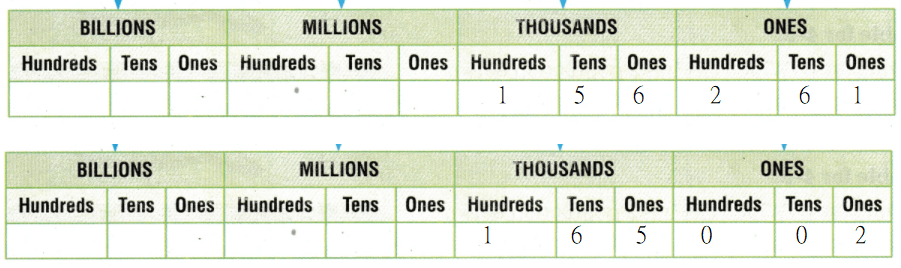Now,
From the above place-value chart,
We can observe that
165,002 > 156,261
Hene, from the above,
We can conclude that
The statement that correctly compares the two mileages is: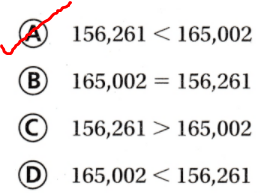Question 10.
Which number is greater than 53,614,293?
(A) 35,828,781
(B) 53,641,192
(C) 53,416,998
(D) 50,967,299
The given number is: 53,614,293
Hence, from the above,
We can conclude that
The number that is greater than 534,614,293 is:Question 11.
Which number makes this statement true?
42,607 > ____________ >40,352
(A) 40,255
(B) 38,976
(C) 42,875
(D) 42,410
Let the missing number be: x
Now
The given pattern is:
42,607 > x > 40,352
Now,
From the above pattern,
We can observe that
x < 42,607 and x > 40,352
Hence, from the above,
We can conclude that
The number that makes the given statement true is:Question 12.
Which digits can replace theto make a true statement?
6,456 < 6,12 < 6,788
(A) 5, 6
(B) 4, 5, 6
(C) 5, 6, 7
(D) 4, 5, 6, 7
Let the missing digit be: x
Now,
The given pattern is:
6,456 < 6,x12 < 6,788
Now,
From the above pattern,
We can observe that
The Hundred’s place-value is increasing
So,
The missing digit should be: 5 (or) 6
Hence, from the above,
We can conclude that
The digits that can replace theto make a true statement are:Question 13.
Multi-Step At Ted’s Used Cars, the sales staff set a goal of $25,500 in sales each week. The sales for three weeks were$28,288; $25,369; and$25,876. Which total did not meet the goal?
(A) $25,500 (B)$28,288
(C) $25,369 (D)$25,876
It is given that
At Ted’s Used Cars, the sales staff set a goal of $25,500 in sales each week. The sales for three weeks were$28,288; $25,369; and$25,876
Now,
The given numbers are: 28,288, 25,369, and 25,876
Now,
Compare the given numbers with 25,500
So,
25,500 < 28,888
25,500 > 25,369
25,200 < 25,876
Hence, from the above,
We can conclude that
The total that did not meet the goal is:Question 14.
Multi-Step Over three years a factory produced 3,516,235; 2,965,005 and 3,261,636 cans of soup. Which lists the numbers in order from least to greatest?
(A) 2,965,005; 3,261,636; 3,516,235
(B) 3,516,235; 3,261,636; 2,965,005
(C) 2,965,005; 3,516,235; 3,261,636
(D) 3,261,636; 2,965,005; 3,516,235
It is given that
Over three years a factory produced 3,516,235; 2,965,005 and 3,261,636 cans of soup
Now,
The given numbers are: 3,516,235, 2,965,005, and 3,261,636
Now,
The representation of the given numbers in the place-value chart is: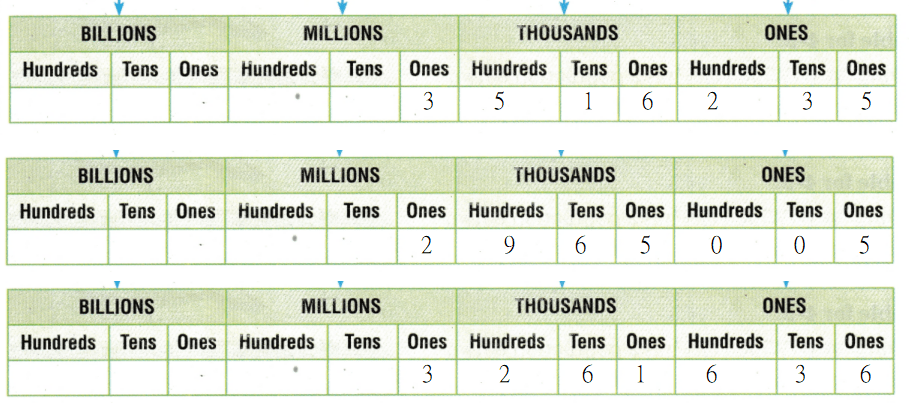Now,
From the above place-value chart,
We can observe that
3,516,235 > 3,261,636 > 2,965,005
Hence, from the above,
We can conclude that
The order of the numbers from the least to the greatest is: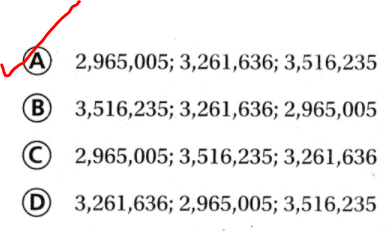Scroll to Top